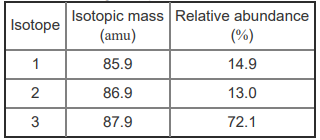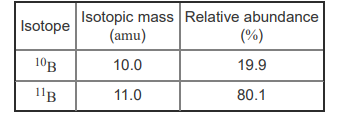# Problem: What is the relative atomic mass of a hypothetical element that consists of the following isotopes in the indicated natural abundances?Express your answer to three significant figures and include the appropriate units.Most elements occur naturally as a mix of different isotopes. The atomic weight, also called the relative atomic mass, of an element is the weighted average of the isotope masses. In other words, it is an average that takes into account the percentage of each isotope. For example, the two naturally occurring isotopes of boron are given here.The relative atomic mass of boron is calculated as follows: (10.0 × 0.199) + (11.0 × 0.801) = 10.8 amuBecause the heavier isotope is more abundant, the relative atomic mass is closer to 11 amu than it is to 10 amu.

###### FREE Expert Solution
100% (449 ratings)View Complete Written Solution
###### Problem Details

What is the relative atomic mass of a hypothetical element that consists of the following isotopes in the indicated natural abundances?Express your answer to three significant figures and include the appropriate units.

Most elements occur naturally as a mix of different isotopes. The atomic weight, also called the relative atomic mass, of an element is the weighted average of the isotope masses. In other words, it is an average that takes into account the percentage of each isotope. For example, the two naturally occurring isotopes of boron are given here.The relative atomic mass of boron is calculated as follows:

(10.0 × 0.199) + (11.0 × 0.801) = 10.8 amu

Because the heavier isotope is more abundant, the relative atomic mass is closer to 11 amu than it is to 10 amu.

Frequently Asked Questions

What scientific concept do you need to know in order to solve this problem?

Our tutors have indicated that to solve this problem you will need to apply the Atomic Mass concept. You can view video lessons to learn Atomic Mass. Or if you need more Atomic Mass practice, you can also practice Atomic Mass practice problems.

What professor is this problem relevant for?

Based on our data, we think this problem is relevant for Professor Ervin's class at UNR.